# Inter mathematics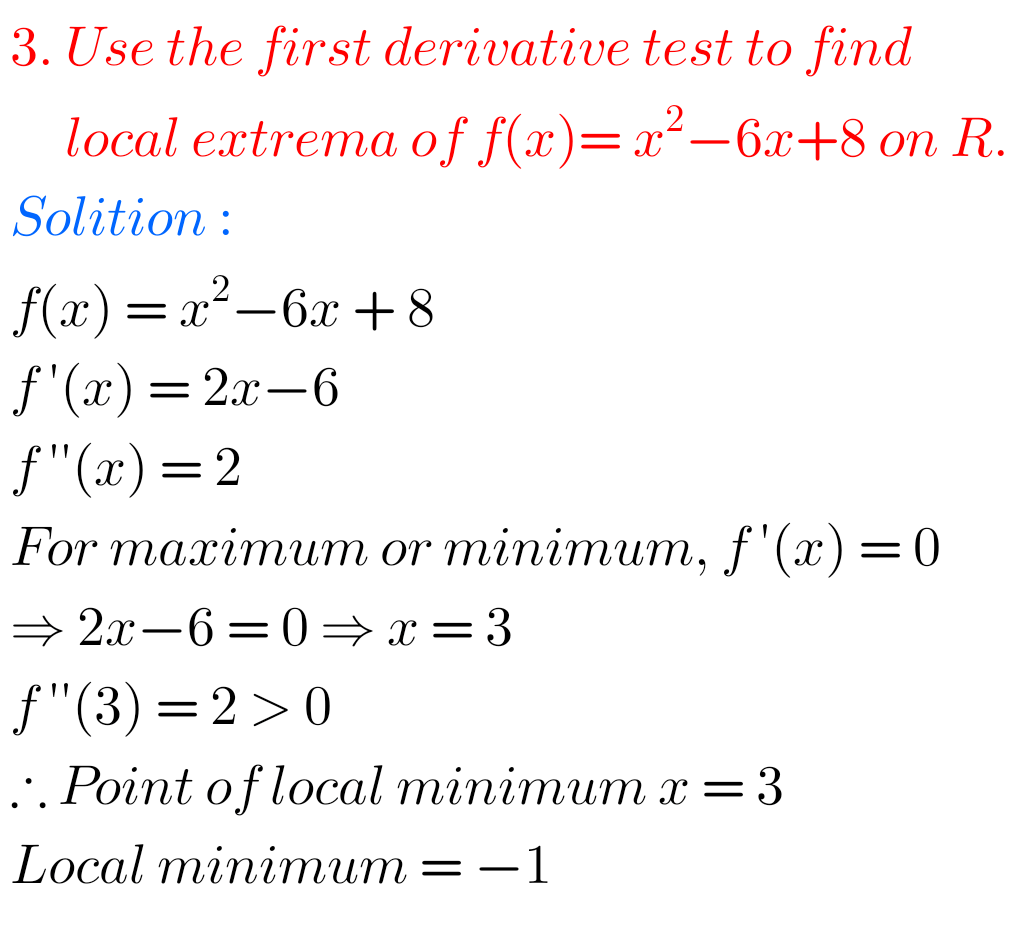## Inter Maths solutions textbook

Intermediate Maths solutions Inter maths textbook solutions are for all chapters are given. Study the textbook lessons very well. Observe the given solutions and try them in your own method. Inter maths IA, IB,IIA and IIB textbook solutions are given Mathematics Inter Solutions Inter Maths 1A textbook solutions Functions Exercise 1(a) Exercise 1(b) Exercise 1(c) …

## Intermediate maths solutions for 2b

Inter maths solutions for 2b Intermediate mathematics IIB textbook exercises solutions are given. Circle, System of circles, Parabola, Ellipse, Hyperbola, Integration, Definite  Integrals and Diffential Equations solutions links are given below. These solutions are very easy to understand. Please study the textbook well.  Observe the given solutions and try them in yuor own method. Intermediate …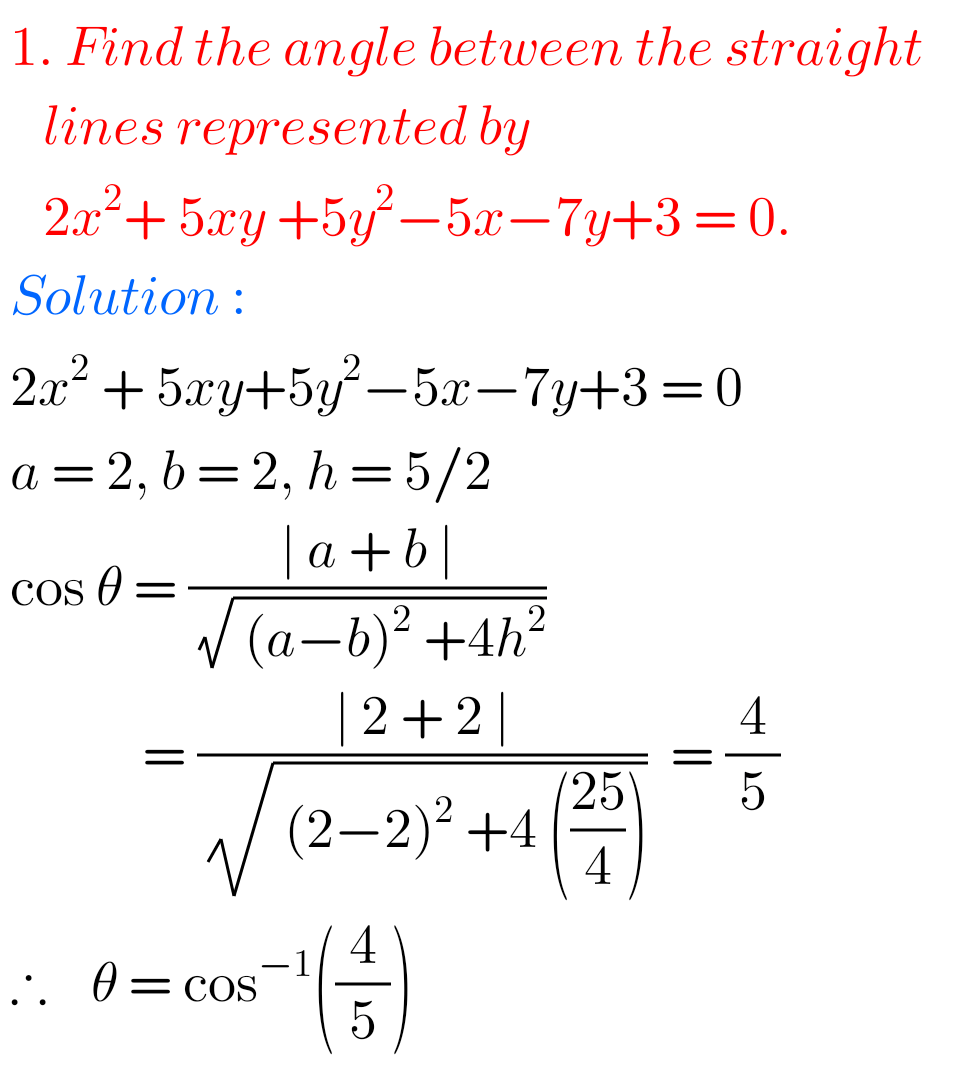## Inter Maths Solutions for Pair of Straight Lines exercise 4(b)

Pair of Straight Lines exercise 4(b) solutions Intermediate maths 1B Pair of Straight Lines exercise 4(b) solutions are given. Study the textbook lesson Pair of Straight Lines very well. Observe the example problems and solutions given in the textbook. Observe the given below solutions and try them in your own method. You can also see …## Chapter 10 exercise 10(h) Applications of Derivatives Inter Maths 1B

Inter Maths Solutions for Applications of Derivatives exercise 10(h) Intermediate Mathematics 1B Applications of Derivatives exercise 10(h) textbook solutions are given. Study the lesson Applications of Derivatives very well. Practice the example problems and solutions given in the textbook. Observe the given below solutions and try them in your own method You can also see …## Applications of Derivatives exercise 10(g) solutions Inter

Inter Maths solutions for Applications of Derivatives Intermediate Mathematics 1B Applications of Derivatives exercise 10(g) textbook solutions are given. Study the lesson Applications of Derivatives very well. Practice the example problems and solutions given in the textbook. Observe the given below solutions and try them in your own method. You can also see Inter Maths …## Intermediate Maths 1B Solutions for exercise 10(f) Applications of Derivatives

Applications of Derivatives exercise 10(f) Solutions Inter Intermediate Mathematics 1B Applications of Derivatives exercise 10(f) textbook solutions are given. Study the lesson Applications of Derivatives very well. Practice the example problems and solutions given in the textbook. Observe the given below solutions and try them in your own method You can also see Inter Maths …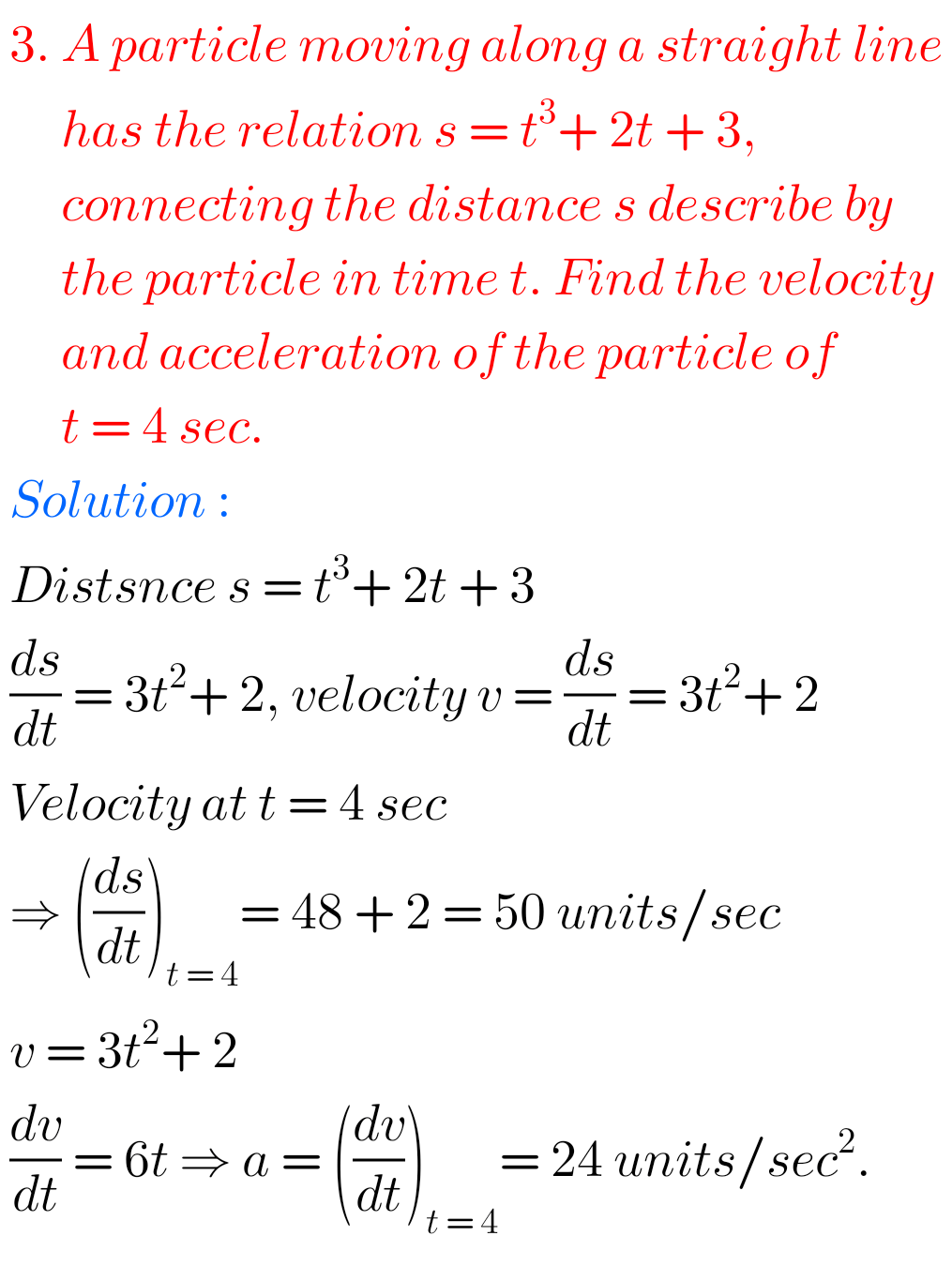## Solutions for Applications of Derivatives exercise 10(e)

Inter Maths 1Bsolutions Applications of Derivatives exercise 10(e) Intermediate Mathematics 1B Applications of Derivatives exercise 10(e) textbook solutions are given. Study the lesson Applications of Derivatives very well. Practice the example problems and solutions given in the textbook Observe the given below solutions and try them in your own method You can also see Inter …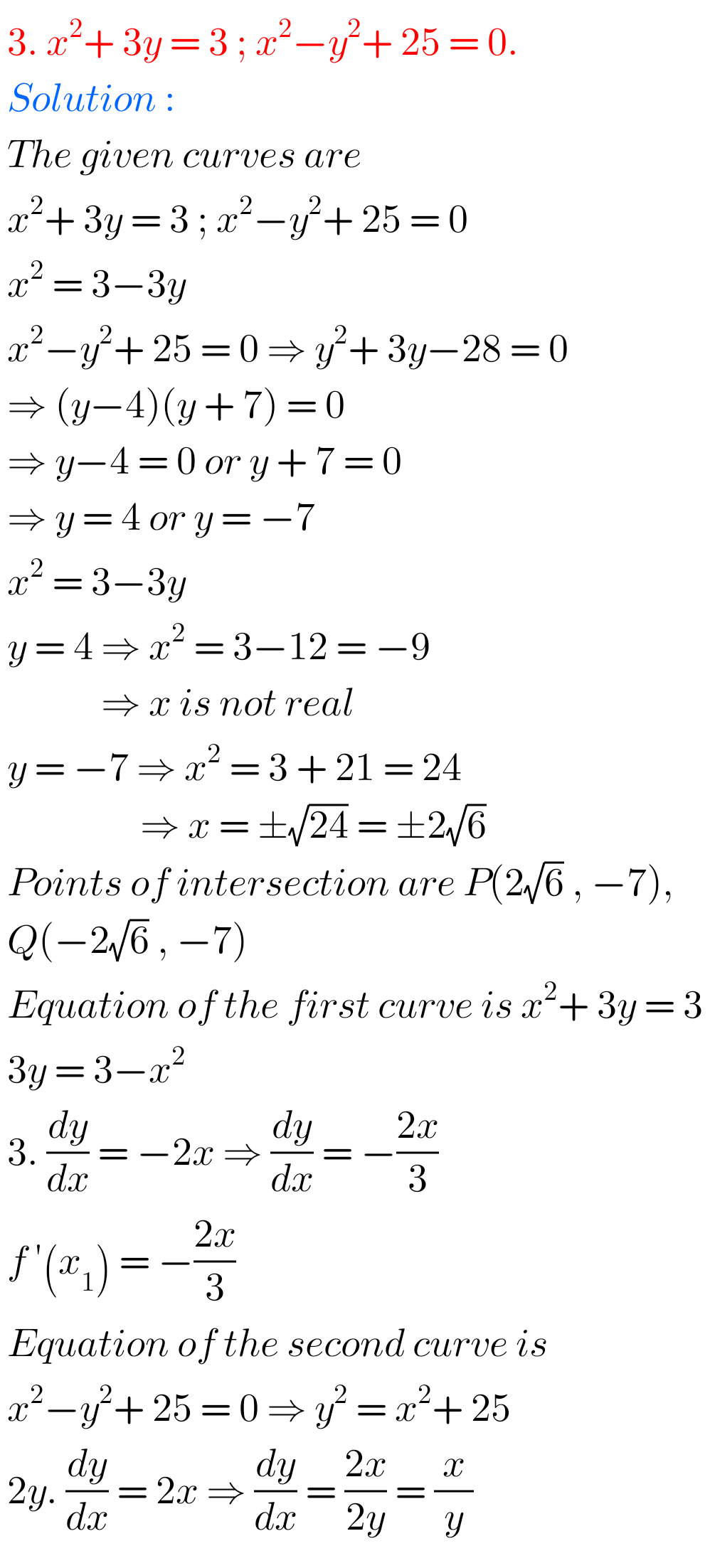## Applications of Derivatives exercise 10(d) solutions Inter

Inter Maths Solutions for Applications of Derivatives exercise 10(d) Intermediate Mathematices 1B Solutions for exercise 10(d) Applications of Derivatives are given. Study the textbook lesson Applications of Derivatives very well. Practice the example problems and solutions given in the textbook. Observe the solutions given below and try them in your own method. You can also …## Exercise 10(c) Applications of Derivatives solutions textbook Inter

Inter Maths solutions for Applications of Derivatives exercise 10(c) Intermediate Mathematices 1B Solutions for exercise 10(c) Applications of Derivatives are given. Study the textbook lesson Applications of Derivatives very well. Practice the example problems and solutions given in the textbook. Observe the solutions given below and try them in your own method. You can also …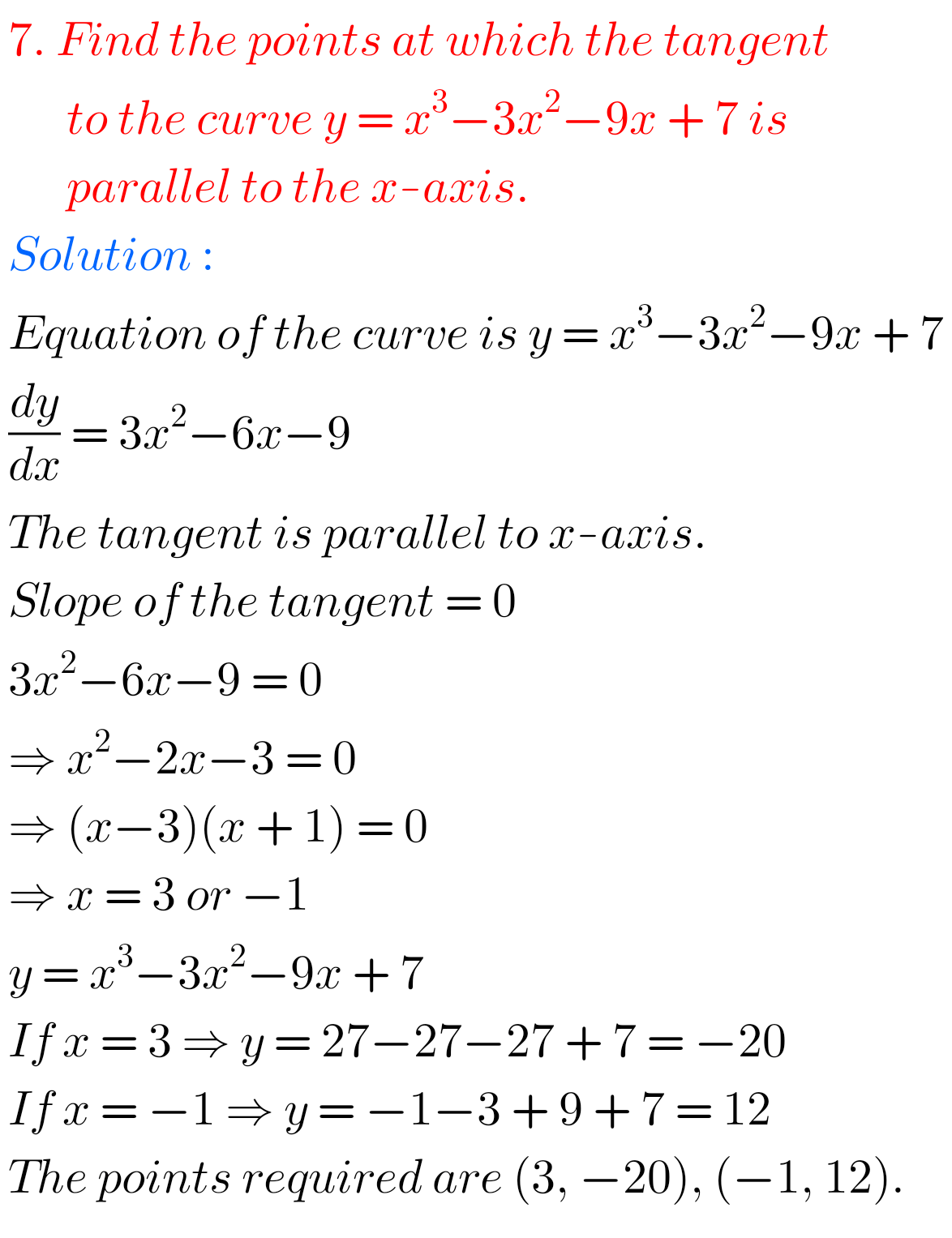## Applications of Derivatives Exercise 10(b) solutions Inter Maths 1B

Intermediate Maths 1B solutions for Applications of Derivatives exercise 10(b) solutions Applications of Derivatives exercise 10(b) Maths 1B textbook Inter solutions are given. Study the textbook lesson Applications of Derivatives very well. Observe the example problems and solutions given in the textbook. Observe the given below solutions and try them in your own method. You …